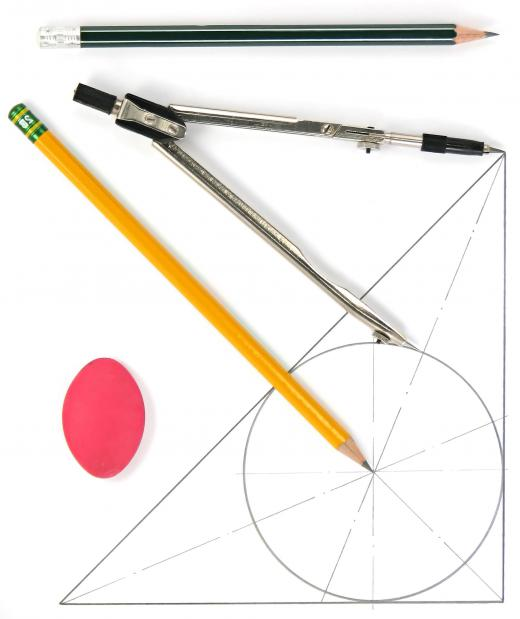Science
Fact Checked

# What Are Geometric Constructions?

E.A. Sanker
E.A. Sanker

Geometric constructions, also called Euclidean constructions after the ancient Greek mathematician Euclid, are geometrically correct figures that are drawn using only a compass and a straightedge. In creating a geometric construction, measurements of angles and lines are not taken, and rulers are not used except as straightedges. This method can be used in drafting technical designs in engineering and as a way of teaching students the fundamentals of geometrical theory.

A drafting compass is an instrument used to draw arcs and circles. It consists of two legs connected by an adjustable center hinge, with one leg ending in a spike and the other holding a pencil lead at its end. The device is used by fixing the spiked end to the paper and inscribing an arc or circle by rotating the pencil end around this fixed center. Circles and arcs of different dimensions can be traced by adjusting the central hinge to a wider or narrower angle.

Straightedges are used in geometric constructions to draw lines and may be any object with a perfectly straight edge. Rulers are often used, though the markings must be ignored in creating the construction. Drafting triangles, which are flat right triangles of plastic or metal used in technical drawing, are another popular choice for a straightedge, although the angles of the triangle should not be used to create the construction.

Many different geometrical figures can be constructed using only the two tools mentioned above. For example, to construct an equilateral triangle, a line segment is first drawn using the straightedge. Suppose this line has endpoints A and B. The compass is fixed at point A and extended so that the pencil lead touches B. An arc is drawn through B to a point above AB.A type of drafting tool, compasses can be used to draw circles and arches of various sizes.

Next, the compass is fixed at point B and another arc is drawn using the same radius, so that the points intersect above line AB. Using the straightedge, a line is drawn from this point of intersection to point A, and another is drawn to point B. The three lines that have been created now form a perfect equilateral triangle.

Geometric constructions are helpful in teaching how geometric figures are related, but they are also used in non-academic settings. Architects and engineers must know the elements of geometric constructions in order to create precise technical drawings for designs of machinery or buildings. Although automated computer-aided design (CAD) systems have replaced manual drawing in most engineering settings, geometric constructions are still widely taught as background information for understanding the principles of design.

## You might also Like